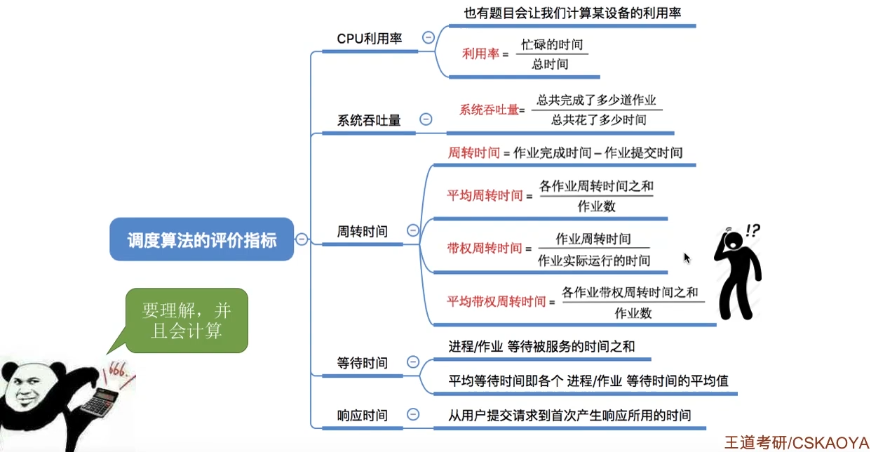# 只言片语

:D 获取中...

#### 1.CPU利用率

CPU利用率：指CPU“忙碌”的时间占总时间的比例

$利用率=\frac{忙碌的时间}{总时间}$

$CPU利用率=\frac{5+5}{5+5+5}=66.665$
$打印机利用率=\frac{5}{15}=33.335$

#### 2.系统吞吐量

$系统吞吐量=\frac{总共完成了多少道作业}{总共花了多少时间}$

$\frac{10}{100}=0.1（道/秒）$

#### 3.周转时间

• 对于用户来说，更关心自己的单个作业的周转时间：

（作业）周转时间 = 作业完成时间 - 作业提交时间

• 对于操作系统来说，更关心系统的整体表现，因此更关心所有作业周转时间的平均值。

$平均周转时间=\frac{各作业周转时间之和}{作业数}$

• 有的作业运行时间长，有的作业运行时间短，因此在周转时间相同的情况下，运行时间不同的作业，给用户的感受肯定是不一样的。

$戴荃周转时间=\frac{作业周转时间}{作业实际运行的时间}=\frac{作业完成时间-作业提交时间}{作业实际运行的时间}$

$平均带权周转时间=\frac{各作业带权周转时间之和}{作业数}$

#### 4.等待时间

$平均等待时间=\frac{所有进程/作业的等待时间之和}{作业数量}$

#### 6.知识回顾